Theorem X (Cauchy Goursat): Suppose that f(z) is analytic everywhere in 𝔻.  Then given 𝛾 ∈ 𝔻,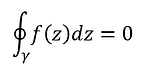Many readers will be inexperienced with proving results such as this.  I am going to walk you through the steps here slowly and carefully.  The kinds of techniques we will use to prove this are ubiquitous in modern mathematics as are the meta-techniques.  The meta-techniques here involve breaking a big proof down into a number of smaller comprehensible pieces.

I will present you with two alternative proofs.  The first will utilize techniques from a prior course.  If we examine a contour and recall that we can express ƒ(z) as u(x, y) + iv(x, y) then this might bring to mind a certain theorem from multivariable calculus: Green's Theorem.  This theorem is among my favorites because it lends itself to endless generalization.  Since u and v are real functions of real variables and

since they are analytic on and in the contour then

we may use Green's theorem presented to the

right.  Try to complete the proof!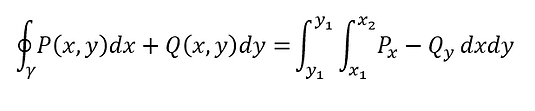Our second idea will focus on more obvious using the tools we have built.

Instead of trying to prove this result for any curve 𝛾, let's first consider a

rectangle with positive orientation.  If we cannot prove this for a rectangle, it seems like it would be pretty hopeless to continue!  Moreover, there is a point to doing it in this manner that might seem beleaguering by the time we finish it: in order to apply Green's Theorem, we had to assume the derivatives were everywhere continuous.  We do not (yet) know this!  We can bake that assumption in, which we did above, but perhaps we have limited ourselves to a much smaller set of functions than we needed to.Recall our fond friend, the ML bound.  Certainly, we can say that

where M is the max of ƒ(z) around the perimeter of the                                  rectangle and L is precisely the perimeter of the rectangle.  Now, we notice that if we subdivide the rectangle into 4 subrectangles of equal area then the integral cancels along the internal subdivisions.  Moreover, one of the rectangles now contains the max of ƒ(z), M1, around those new bounds.  It might be larger than our original M, but we can find it.  We'll say it's on R1, without loss of generality.  (If it were not, we would just renumber our rectangles so that it were so!).  We can further subdivide R1 into 4 equal area rectangles and find from amongst them an R2 where ƒ(z) takes its max.  If we continue to do this, we will notice that at the n  iteration, we have

th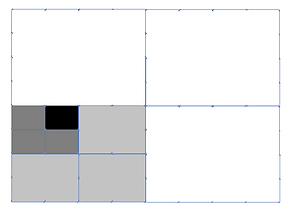th

Now, we will need to use our ML bound for the rightmost integral.  We notice that the L here is just the perimeter of the shrinking rectangle on the n  iteration.  If we call the perimeter of the original rectangle P and notice that it shrinks by half at each step then we find           .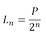We will need a further observation from earlier.  Recall Cantor's Theorem for metric spaces.  We know that our rectangles satisfy the hypotheses of this theorem and therefore must converge to a single point, call it ζ.  Since we know this point exists, that we converging to this point as n grows large, and that ƒ(z) is continuous and differentiable in the neighborhood of ζ, then we can select an n large enough a z near enough to guarantyD here is the length of the diagonal because that is as far as one can get from ζ in a given rectangle.  But, as n gets large, it becomes necessary that that D must shrink proportionally to the perimeter.  Here I'd like to point out we used the hypothesis of differentiability to obtain the first inequality and then we used the fact that Rn is a rectangle with diameter proportional to D multiplied by the scaling factor of the perimeter to obtain the last inequality.  To finish the proof for a rectangle, we begin where we previously left off.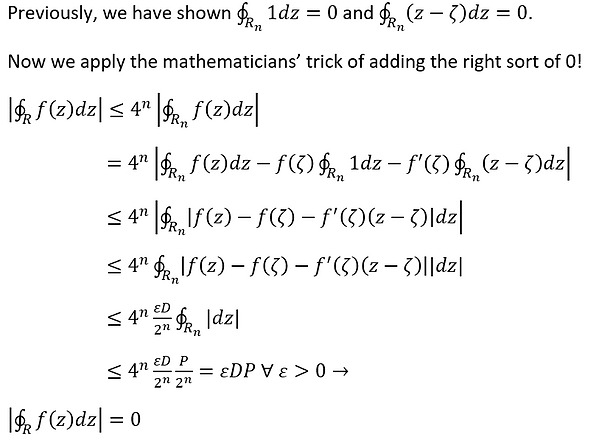Here, we have now proved this statement for all rectangles.  That seems like a far cry from our desired result.  But we had additional assumptions.  Recall that 𝛾 is closed, rectifiable, and simply connected.  Since it is rectifiable I can find a finite curve that is made entirely of uniformly sized line segments that is a good approximation of 𝛾.  Now, I can fill the area bounded by 𝛾 with rectangles of uniform size.  All of the interior segments cancel because they will all be traversed forwards and backwards.

As you notice in the figure on the right, with each iteration, my rectangular gridding becomes closer and closer to 𝛾.  Eventually, it will be within 𝜀 of 𝛾 ∀ 𝜀 > 0.   Every integral of ƒ(z) around the rectangular approximation is zero by the above reasoning, therefore we have established the theorem.  In this second iteration of the proof, we did not assume the partial derivatives were continuous, so in a sense we have arrived at a stronger result.